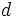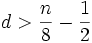# Hopf conjecture

Jump to: navigation, search

## Statement

Any compact, even-dimensional Riemannian manifold with everywhere positive sectional curvature, must have positive Euler characteristic.

## Progress towards the conjecture

### In dimension two

The Hopf conjecture is true in dimension two, following trivially from the Gauss-Bonnet theorem.

### In dimension four

Th Hopf conjecture is also true in dimension 4. The proof idea is as follows. We write down a Gauss-Bonnet formula (which generalizes the Gauss-Bonnet theorem to even-dimensional manifolds) which equates the Euler characteristic to a certain integral over the manifold. We then show that in dimension four, positivity of the sectional curvature implies positivity of the integrand, and hence, of the integral.

(Note that this holds even in dimension two, where the integrand is the sectional curvature upto normalization).

### Why this fails in higher dimensions

In higher dimensions, positivity of the sectional curvature no longer implies positivity of the integrand. It may still be true, though, that the overall integral remains positive.

### Partial proof for higher dimensions

It turns out that the Hopf conjecture is true on the manifold if it admits a$d$-dimensional isometric torus action, where:$d > \frac{n}{8} - \frac{1}{2}$